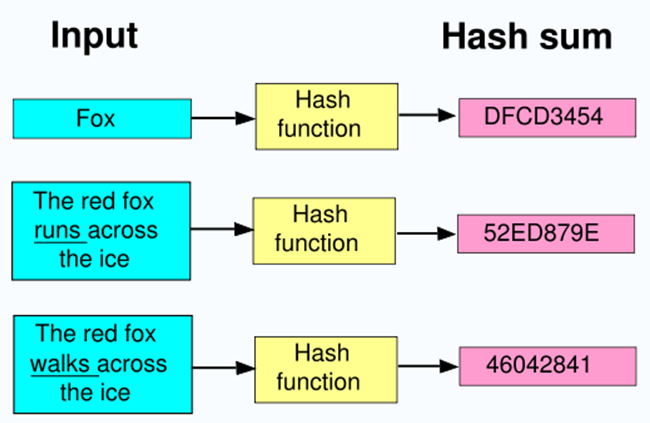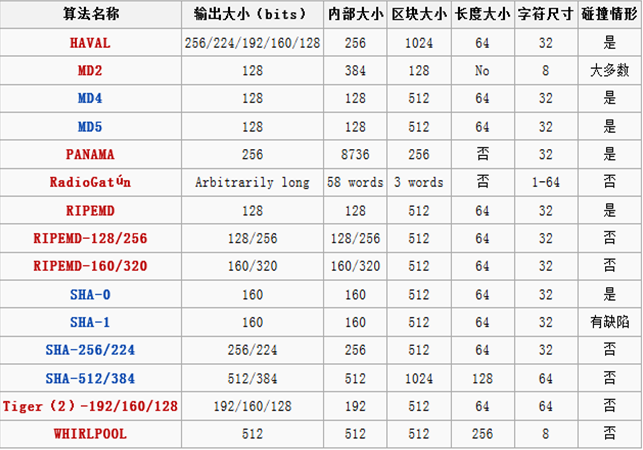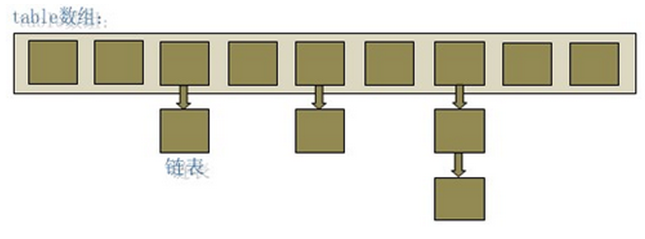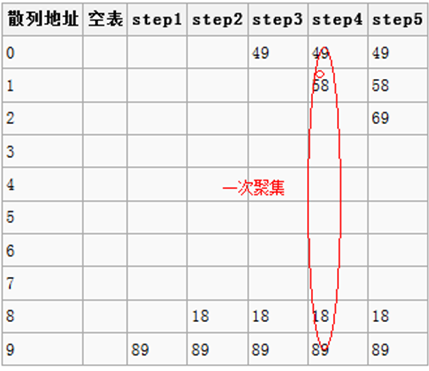Java数据结构之散列表(动力节点Java学院整理)

1、加密散列，在信息安全领域使用

2、散列表，一种使用散列函数将键名和键值关联起来的数据结构

3、关联数组，一种常常使用散列表来实现的数据结构

4、几何散列，寻找相同或相似的几何形状的一种有效方法``` /**
* Retrieve object hash code and applies a supplemental hash function to the
* result hash, which defends against poor quality hash functions. This is
* critical because HashMap uses power-of-two length hash tables, that
* otherwise encounter collisions for hashCodes that do not differ
* in lower bits. Note: Null keys always map to hash 0, thus index 0.
*/
final int hash(Object k) {
int h = 0;
if (useAltHashing) {
if (k instanceof String) {
return sun.misc.Hashing.stringHash32((String) k);
}
h = hashSeed;
}
h ^= k.hashCode();
// This function ensures that hashCodes that differ only by
// constant multiples at each bit position have a bounded
// number of collisions (approximately 8 at default load factor).
h ^= (h >>> 20) ^ (h >>> 12);
return h ^ (h >>> 7) ^ (h >>> 4);
} ```1、直接地址法：

Tips： 简单的思考一下这种方式就可以知道，这种方式基本不会存在哈希冲突，不过事先我们应该知道key集合的大小，而且使用线性函数值作为散列地址的话，很大程度上造成了空间的浪费。hash(k)=k这种方式更加的鸡肋没必要，以这种方式散列还不如直接数组索引。

2、数字分析法：

Tips：这种方式极度不灵活，限制太多。

3、平方取中法：

Tips：这种方式中间的几位数都和关键字的没一位都有关，产生的散列地址较为的均匀。

4、折叠法:

5、除留余法：

6、随机法：

h(key)=random(key)??? 其中random为伪随机函数，但要保证函数值是在0到m-1之间。总结:在上述的方法中，3、4、5三种方法的结合使用方式较好，在JDK以前的版本就是使用的方法5。

1、分离连接法：HashMap中冲突处理代码如下???

```for (Entry<K,V> e = table[i]; e != null; e = e.next) {
Object k;
if (e.hash == hash && ((k = e.key) == key || key.equals(k))) {
V oldValue = e.value;
e.value = value;
e.recordAccess(this);
return oldValue;
}
} ```

2、开放地址法

hash_i=(hash(key) + d(i)) mod m, i=1,2...k\,(k < m-1)，其中hash(key)为散列函数，m为散列表长，d(i)为增量序列，i为已发生碰撞的次数。增量序列可有下列取法：

d(i)=1,2,3...(m-1) 称为 线性探测；即 d(i)=i ，或者为其他线性函数。相当于逐个探测存放地址的表，直到查找到一个空单元，把散列地址存放在该空单元。d(i)=1^2,? 2^2,3^2... k^2 (k < m/2) 称为 平方探测。相对线性探测，相当于发生碰撞时探测间隔 d(i)=i^2 个单元的位置是否为空，如果为空，将地址存放进去。d(i)=伪随机数序列，称为 伪随机探测。1、开始时hash(89)=9无冲突，直接插入；

2、hash(18)=8无冲突，直接插入；

3、hash(49)=9冲突了，开放地址，将49放入下一个空闲地址0

4、hash(58) =8冲突了，开放地址，将58放入9冲突 ，放入0冲突、放入1

5、hash(69) =9冲突，开放地址，将69放入0冲突，放入1冲突，放入2

Tips：思考其缺点!

3、双散列、再散列